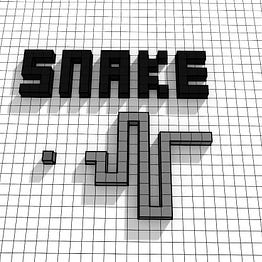# Graph Traversal in Python: BFS,DFS,Dijkstra,A-star parallel comparision

We have discussed about Breadth First Search (BFS), Depth First Search (DFS), Dijkstra’ Search, A-star (or A*) algorithm. Let’s do a quick review and comparision of all the past 4 articles introducing Graph Traversal algorithm. This will be the last article about graph traversal.

Briefly, BFS and DFS just go through all the nodes with their own preference. One goes broadwise and the other dig deeper. Whereas Diskstra looks smarter and able to pick a smaller step for its preference. A* cheated by getting answer from user.

In a single case, which one is more efficient is all by luck…

# Graph Traversal in Python:A* algorithm

We have gone through Breadth First Search (BFS), Depth First Search (DFS), Dijkstra’s Search in Python previously. In this articles we will go through the A* algorithm with a few examples and illustration. Then we will try to compare these algorithms in parallel.

Briefly, BFS and DFS are literally traversal around all the node in the entire graph with no particular “destination ”or “goal”. Dijkstra did the same but its destination is “go everywhere” (all node).

These are not what we want because intutively a search algorithm should start from somewhere and end somewhere, point to point. …

# Graph Traversal in Python: Dijkstra’s Search

Previously we have gone through the most fundamental graph search algorithm: the Breadth First Search (BFS) and the Depth First Search (DFS). The two basic algorithms share one common point that their edges have no weights. What are weights? Simply speaking they are the “road length” or “distance between two cities”.

Weights of graph exactly reflect the real world spatial domain, though they can represent more abstract situation if necessary. But for now, a “map model” is sufficient enough for us to understand a graph and graph traversal. A graph without and with weights can be seen as illustration below…

# Graph Traversal in Python: Depth First Search (DFS)

Depth First Search (DFS) or Depth First Traversal (DFT) is another fundamental graph algorithm that similar to the previous discussed BFS or BFT. The only, minor difference is that the depth first algorithm goes into deeper level node whenever it’s possible.

For example, in the same case as BFS starting with node “A”, DFS goes to “B” and followed by “D” and “E”, “F”. Then goes to “C”. But why it scans “E” after “D” , arn’t “E” and “D” at the same level? That is because “D” has no more subnode connected. The algorithm goes back to “B” and…

# Graph Traversal in Python: Breadth First Search (BFS)

Breadth First Search (BFS), also named as Breadth First Traversal (BFT), is one of the most fundamental graph traversal algorithms. These algorithms are widely used in logistic/delivery routing, map routing, maze path finding and so on.

The so called “breadth” is something illustrated like below: you scan B and C first but not B->D or B->E or B->F or C->F. Before you finish the “shallow layer” of the graph you can’t go further deep to other vertexs. (If you go deep first, that would be the Depth First Search)

Sepcifically, B and C are the direct subnode of A. The…know nothing